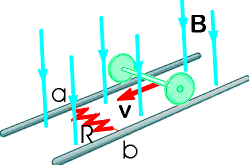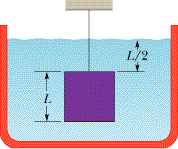Ask question

# Three long wires (wire 1, wire 2,and wire 3) are coplanar and hang vertically. The distance betweenwire 1 and wire 2 is 16.0 cm. On theleft, wire 1 carries an upward current of 1.50 A. To the right,wire 2 carries a downward current of 3.40 A. Wire 3 is located such that when itcarries a certain current, no net force acts upon any of the wires. (a) Find the position of wire 3, relative to wire 1. (b) Find the magnitude and direction of the current in wire 3.# Three long wires (wire 1, wire 2,and wire 3) are coplanar and hang vertically. The distance betweenwire 1 and wire 2 is 16.0 cm. On theleft, wire 1 carries an upward current of 1.50 A. To the right,wire 2 carries a downward current of 3.40 A. Wire 3 is located such that when itcarries a certain current, no net force acts upon any of the wires. (a) Find the position of wire 3, relative to wire 1. (b) Find the magnitude and direction of the current in wire 3.

Question
Otherasked 2021-03-15
Three long wires (wire 1, wire 2,and wire 3) are coplanar and hang vertically. The distance betweenwire 1 and wire 2 is 16.0 cm. On theleft, wire 1 carries an upward current of 1.50 A. To the right,wire 2 carries a downward current of 3.40 A. Wire 3 is located such that when itcarries a certain current, no net force acts upon any of the wires.
(a) Find the position of wire 3, relative to wire 1.
(b) Find the magnitude and direction of the current in wire 3.

## Answers (1)2021-03-17
Force between two current carrying wires( wire 1 and wire 2)
$$\displaystyle{F}_{{{12}}}={\frac{{{K}{i}_{{1}}{i}_{{2}}}}{{{2}\pi{r}}}}$$
as both the wires carrying currents in opposite directions thenature of the fore on 2nd due to 1st is repulsive. hence to nullify the net force acting on the second conductorthird conductor should carry current in upward direction.
Then again 2nd and 3rd conductors carrying currents inopposite directions. hence again 2nd capacitor will experiencerepusive force.
If both the forces are equal then net force acting on thesecond is zero.
$$\displaystyle{F}_{{{23}}}={\frac{{{K}{i}_{{2}}{i}_{{3}}}}{{{2}\pi{x}}}}$$
x is distance
given net force should be zero, hence $$\displaystyle{F}_{{{12}}}={F}_{{{23}}}$$
$$\displaystyle{\frac{{{i}_{{1}}{i}_{{2}}}}{{{2}\pi{r}}}}={\frac{{{i}_{{2}}{i}_{{3}}}}{{{2}\pi{x}}}}$$
$$\displaystyle{i}_{{1}}={1.50}{A};{i}_{{2}}={3.40}{A};{r}={0.16}{m}$$
similarly calculate the net force on the third due to 1st and2nd and following the same procedure we get
$$\displaystyle{\frac{{{i}_{{1}}{i}_{{3}}}}{{{\left({16}+{x}\right)}\times{10}^{{-{12}}}}}}={\frac{{{i}_{{2}}{i}_{{3}}}}{{{x}}}}$$
so we have two unknowns and two equations try to calculatex and $$\displaystyle{i}_{{3}}$$; the directions of current $$\displaystyle{i}_{{3}}$$ is upwards as mentioned above

### Relevant Questionsasked 2021-03-30
A long, straight, copper wire with a circular cross-sectional area of $$\displaystyle{2.1}{m}{m}^{{2}}$$ carries a current of 16 A. The resistivity of the material is $$\displaystyle{2.0}\times{10}^{{-{8}}}$$ Om.
a) What is the uniform electric field in the material?
b) If the current is changing at the rate of 4000 A/s, at whatrate is the electric field in the material changing?
c) What is the displacement current density in the material in part (b)?
d) If the current is changing as in part (b), what is the magnitude of the magnetic field 6.0cm from the center of the wire? Note that both the conduction current and the displacement currentshould be included in the calculation of B. Is the contribution from the displacement current significant?asked 2021-05-03
A charge of $$\displaystyle{6.00}\times{10}^{{-{9}}}$$ C and a charge of $$\displaystyle-{3.00}\times{10}^{{-{9}}}$$ C are separated by a distance of 60.0 cm. Find the position at which a third charge, of $$\displaystyle{12.0}\times{10}^{{-{9}}}$$ C, can be placed so that the net electrostatic force on it is zero.asked 2021-03-21
In the figure below, the rolling axle, 1.43 m long, is pushed along horizontal rails at a constant speed v = 3.36 m/s.A resistor R = 0.325 ohm is connected to the rails at points a and b, directly opposite each other. (The wheels make good electrical contact with the rails, and so the axle, rails, and R form a closed-loop circuit. The only significant resistance in the circuit is R.) There is a uniform magnetic field B = 0.0850 T vertically downward. Calculate the induced current I in the resistor and what horizontal force F is required to keep the axle rolling at constant speed?asked 2021-04-19
In the figure, a cube of edge length L = 0.599 m and mass 970 kg is suspended by a rope in an open tank of liquid of density 1.05E+3 kg/m3. Find (a) the magnitude of the total downward force on the top of the cube from the liquid and the atmosphere, assuming atmospheric pressure is 1.00 atm, (b) the magnitude of the total upward force on the bottom of the cube, and (c) the tension in the rope. (d) Calculate the magnitude of the buoyant force on the cube using Archimede's principle.asked 2021-02-19
A 10 kg objectexperiences a horizontal force which causes it to accelerate at 5 $$\displaystyle\frac{{m}}{{s}^{{2}}}$$, moving it a distance of 20 m, horizontally.How much work is done by the force?
A ball is connected to a rope and swung around in uniform circular motion.The tension in the rope is measured at 10 N and the radius of thecircle is 1 m. How much work is done in one revolution around the circle?
A 10 kg weight issuspended in the air by a strong cable. How much work is done, perunit time, in suspending the weight?
A 5 kg block is moved up a 30 degree incline by a force of 50 N, parallel to the incline. The coefficient of kinetic friction between the block and the incline is .25. How much work is done by the 50 N force in moving the block a distance of 10 meters? What is the total workdone on the block over the same distance?
What is the kinetic energy of a 2 kg ball that travels a distance of 50 metersin 5 seconds?
A ball is thrown vertically with a velocity of 25 m/s. How high does it go? What is its velocity when it reaches a height of 25 m?
A ball with enough speed can complete a vertical loop. With what speed must the ballenter the loop to complete a 2 m loop? (Keep in mind that the velocity of the ball is not constant throughout the loop).asked 2021-03-12
A 75.0-kg man steps off a platform 3.10 m above the ground. Hekeeps his legs straight as he falls, but at the moment his feettouch the ground his knees begin to bend, and, treated as aparticle, he moves an additional 0.60 m before coming torest.
a) what is the speed at the instant his feet touch theground?
b) treating him as a particle, what is his acceleration(magnitude and direction) as he slows down, if the acceleration isassumed to be constant?
c) draw his free-body diagram (see section 4.6). in termsof forces on the diagram, what is the net force on him? usenewton's laws and the results of part (b) to calculate the averageforce his feet exert on the ground while he slows down. expressthis force in newtons and also as a multiple of his weight.asked 2021-05-20
Assume that a ball of charged particles has a uniformly distributednegative charge density except for a narrow radial tunnel throughits center, from the surface on one side to the surface on the opposite side. Also assume that we can position a proton any where along the tunnel or outside the ball. Let $$\displaystyle{F}_{{R}}$$ be the magnitude of the electrostatic force on the proton when it islocated at the ball's surface, at radius R. As a multiple ofR, how far from the surface is there a point where the forcemagnitude is 0.44FR if we move the proton(a) away from the ball and (b) into the tunnel?asked 2021-05-06
Two uniformly charged spheres are firmly fastened to andelectrically insulated from frictionless pucks on an airtable. The charge on sphere 2 is three times the charge onsphere 1. Draw the force diagram that correctly showsthe magnitude and direction of the electrostatic forces. Explain your reasoning.asked 2021-03-24
A man with mass 70.0 kg stands on a platform withmass 25.0 kg. He pulls on the free end of a rope thatruns over a pulley on the ceiling and has its other end fastened tothe platform. The mass of the rope and the mass of the pulley canbe neglected, and the pulley is frictionless. The rope is verticalon either side of the pulley.
a) With what force does he have to pull so that he and theplatform have an upward acceleration of $$\displaystyle{1.80}\frac{{m}}{{s}^{{2}}}$$?
b) What is the acceleration of the rope relative to him?asked 2021-04-24
Problem: From what maximum height can a 75 kg person jump w/obreaking the lower leg bone on either leg? Ignore air resistance and assume the CM of the person moves a distance of 0.60m from the standing to the seat position (that is, in breaking the fall). Assume the breaking strength (force per unit area) of bone is $$\displaystyle{170}\times{10}^{{6}}\ \frac{{N}}{{m}^{{2}}}$$ and its smallest cross sectional areais $$\displaystyle{2.5}\times{10}^{{-{4}}}$$
...